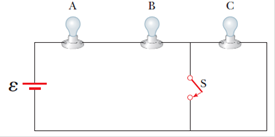# A circuit consists of three identical lamps, each of resistance R, connected to a battery as in Figure P18.51. (a) Calculate an expression for the equivalent resistance of the circuit when the switch is open. Repeat the calculation when the switch is closed. (b) Write an expression for the power supplied by the battery when the switch is open. Repeat the calculation when the switch is closed. (c) Using the results already obtained, explain what happens to the brightness of the lamps when the switch is closed.FIGURE P18.51

Question

A circuit consists of three identical lamps, each of resistance R, connected to a battery as in Figure P18.51. (a) Calculate an expression for the equivalent resistance of the circuit when the switch is open. Repeat the calculation when the switch is closed. (b) Write an expression for the power supplied by the battery when the switch is open. Repeat the calculation when the switch is closed. (c) Using the results already obtained, explain what happens to the brightness of the lamps when the switch is closed.

FIGURE P18.51### Want to see this answer and more?

Experts are waiting 24/7 to provide step-by-step solutions in as fast as 30 minutes!*

*Response times may vary by subject and question complexity. Median response time is 34 minutes for paid subscribers and may be longer for promotional offers.
Tagged in
Science
Physics

### Other